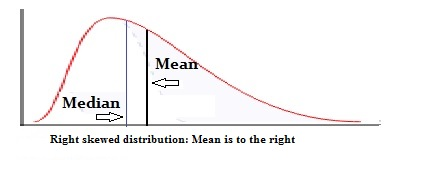# Moment

## What is a Moment?

A moment is a quantitative measurement for the shape of a function. Moments are applied in both mechanics and mathematics as ways of describing distributions and are defined by the function:

sth moment = (x1s + x2s + x3s + . . . + xns)/n

The first moment, where the variable in the function is replaced with value 1, produces the function's mean. Similarly, the second moment, also known as the central moment, defines the function's variance. Additionally, the third moment produces the function's skewness, or the extent to which the probability distribution is offset from the mean. The fourth moment generates a function's kurtosis, which is defined as the shape of the tail of a probability distribution. Like the third moment that describes skewness, the fourth moment is normalized, meaning the moment function is divided by an expression of the standard deviation. This allows for the third and fourth moments to be scale invariant.Source

## How does a Moment work?

As described mathematically above, Moments define aspects of a distribution. Generally, Moments provide a straightforward method for understanding a data set's variance, mean, skewness, etc. However, sometimes it is necessary to find the mean and variance of a random variable, and this proves to be a little more challenging. For this scenario, it is helpful to use a moment-generating function